### Home > A2C > Chapter 12 > Lesson 12.4.1 > Problem12-169

12-169.
1. Explain each transformation below in terms of the parent graph y = tanθ. Homework Help ✎

1. y = tanθ + 1

2.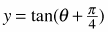3. y = −tan(θ)

4. y = 4 tanθ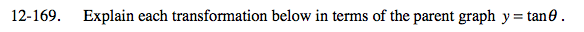For the following questions, compare the equations to the parent y = tan(x).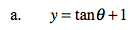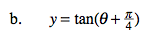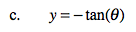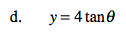Use the eTool below to explore the transformations.
Click the link at right for the full version of the eTool: A2C 12-169 HW eTool